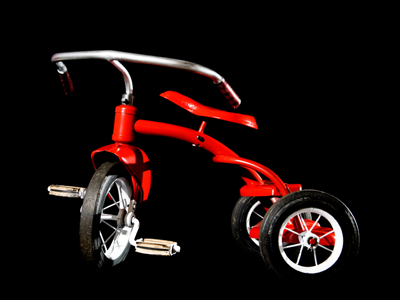A tricycle has three wheels. Can you think of other things that come in threes?

# 11 Times Table

If you are an 11-plus student, then you will need no introduction to the times tables. If you aren't hugely confident with your times tables, then you might want to brush up your knowledge by playing this quiz which looks at the eleven times table.

Learning the eleven times table is all about multiplying numbers by 11. For smaller numbers it's easy to do this - just write the number twice: 3 x 11 = 33, 7 x 11 = 77. When it comes to double digit numbers though, things get a little harder. Keep in mind the much easier 10 times table and that should help. First multiply the number by 10 and then add the original number.

You may think that you've cracked the times tables by now - but be on your guard! Overconfidence can cause carelessness and carelessness leads to mistakes. Take your time and choose each answer wisely!

Keep coming back to these times table quizzes even when you've mastered them. Practise makes perfect and it will help to make the times tables second nature.

1.
4 x 11 =
33
44
48
52
44 is the telephone code for international calls to the United Kingdom
2.
11 x 7 =
77
78
87
88
10 x 7 = 70 and 70 + 7 = 77
3.
11 x 12 =
121
129
132
136
To work this one out, first multiply 12 x 10 (120), then add 12 (132)
4.
8 x 11 =
80
81
85
88
In bingo, the number 88 is known as two fat ladies!
5.
11 x 5 =
50
55
58
65
In the USA the speed limit is 55mph on most highways
6.
9 x 11 =
91
99
111
119
A lot of shops price their goods ending with 99 because 99p sounds a lot less than a pound!
7.
11 x 6 =
66
67
68
69
England won the football World Cup in 1966
8.
3 x 11 =
27
29
31
33
There are plenty of things that come in threes. Most clovers have 3 leaves, but if you're very lucky you might find a 4-leafed clover
9.
2 x 11 =
18
20
21
22
To multiply a number by 2 simply add it to itself
10.
11 x 11 =
111
117
121
122
The number 11 is known as 'legs eleven' in bingo because the numbers looks like a pair of legs!
Author:  Sarah Garratty